Next: 6.3.2 CML Inverter Up: 6.3 Examples Previous: 6.3 Examples

## 6.3.1 Output Stage of an OpAmp

A simplified schematic of the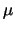A709 operational amplifier output stage is shown in Fig. 6.8. Transistor T3 acts as a common-emitter driver stage for the complementary output devices T1 and T2. Problems are caused by the large bias voltages of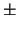15 V and by the sensitivity of the circuit to the state of transistor T3. The operating point is calculated for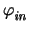= - 14.5 V and from this pointis stepped until -14.1I>V. Within this interval the internal state of the circuit changes completely as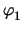moves from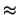13.6 V down to- 15 V. Withthe states of the two output transistors change.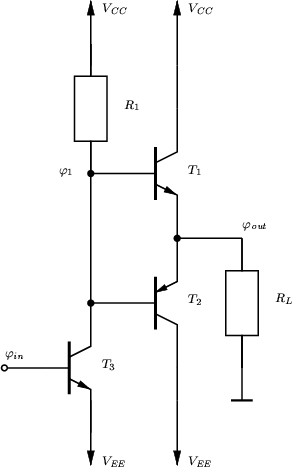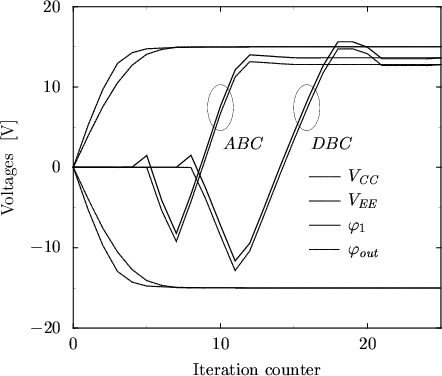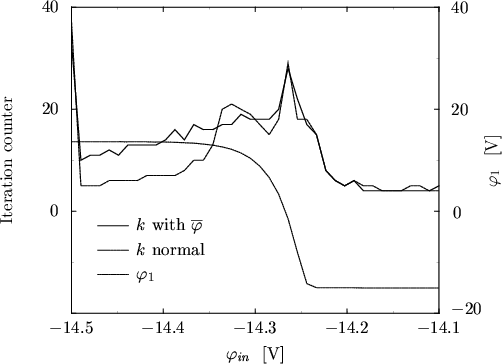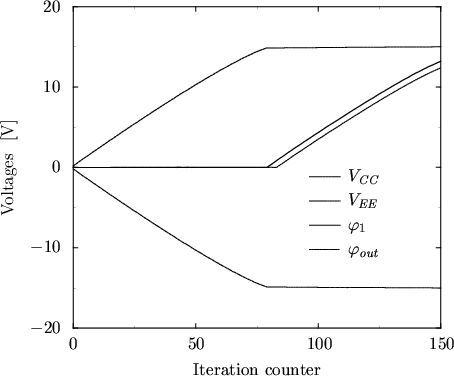The evolution of the node voltages during DC operating point calculation is shown in Fig. 6.9. Best results were obtained with= 4. With ABC 34 iterations were needed and KA = 34/30 = 1.13 whereas with DBC 38 iterations were needed and KD = 38/31 = 1.226.

For the DC transfer characteristic the required number of iterations is shown in Fig. 6.10. In additionis shown to represent the internal state of the circuit. In this case DBC is superior compared to ABC.

To demonstrate the importance of GSk the evolution of the node voltages for a constant GSk = 1/Gmin is shown in Fig. 6.11. To obtain convergence at all, the global damping parameterhad to be increased by a factor of 100 and 170 iterations were necessary.

Although ABC required less iterations than DBC this type of boundary condition seems to have a negative impact on the condition of the system matrix as each contact voltage depends on the node voltages of all other contacts. Since an iterative solver is used which is very sensitive to the condition of the matrix the total simulation time is unfortunately larger than for DBC.Next: 6.3.2 CML Inverter Up: 6.3 Examples Previous: 6.3 Examples
Tibor Grasser
1999-05-31